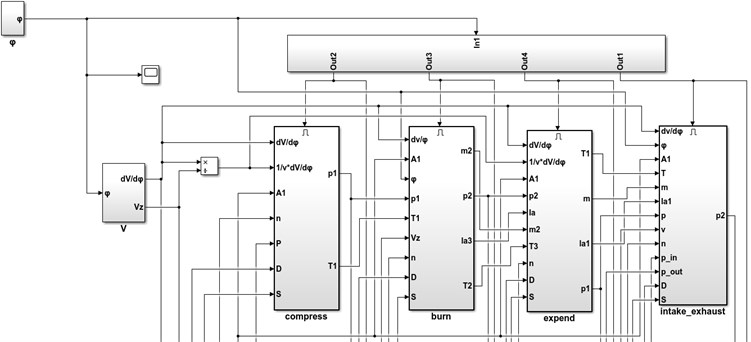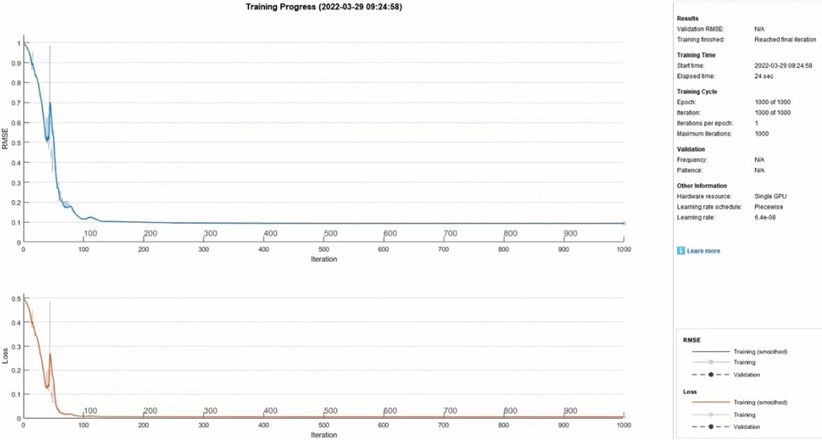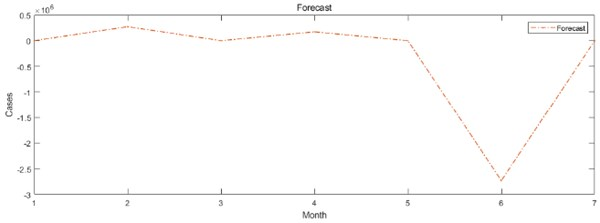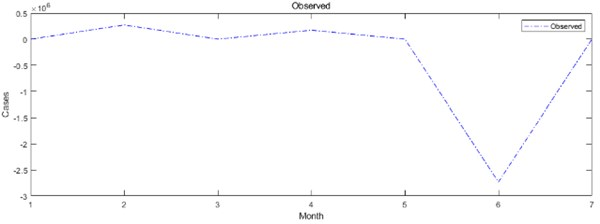# Thermal fault diagnosis of marine diesel engine based on LSTM neural network algorithm

## Yu Zhao1, Shuheng Wang2, Ning Chen3

1, 2, 3School of Energy and Power, Jiangsu University of Science and Technology, Zhenjiang, China

1Corresponding author

Vibroengineering PROCEDIA, Vol. 41, 2022, p. 198-203. https://doi.org/10.21595/vp.2022.22515
Received 15 March 2022; received in revised form 29 March 2022; accepted 8 April 2022; published 21 April 2022

55th International Conference on Vibroengineering, Almaty, Kazakhstan, April 21, 2022

Copyright © 2022 Yu Zhao, et al. This is an open access article distributed under the Creative Commons Attribution License, which permits unrestricted use, distribution, and reproduction in any medium, provided the original work is properly cited.
Views 67
CrossRef Citations 0
Abstract.

As the power source of the ship sailing, diesel engine will inevitably produce some thermal faults in the course of sailing, which will affect the stability of the ship sailing, so the diagnosis of thermal faults becomes very important. This paper uses Simulink software platform to simulate the thermal failure of diesel engine, and selects seven thermal parameters as the source of data set. The data set is input into LSTM neural network algorithm diagnosis model, several typical fault modes of diesel engine are output, and the data processing and image drawing are carried out in Matlab. Compared with other algorithms, LSTM neural network algorithm solves the long time dependence problem and has a high interpretation of the predicted data. The results show that the fault diagnosis model based on LSTM neural network algorithm can diagnose the diesel engine fault mode well.

Keywords: diesel engine, thermal failure, Simulink, LSTM neural network, fault diagnosis.

#### 1. Introduction

Some faults may occur in marine diesel engine during actual navigation. Once these faults occur, they will cause abnormal thermal parameters and eventually lead to the decline of the performance index of the diesel engine. These faults are usually referred to as thermal failures . Barell L. et al. studied the vibration test method of the cylinder head of the internal combustion engine, and proved that the vibration of the cylinder head had a strong corresponding relationship with the working conditions in the cylinder. Therefore, the working conditions of the internal combustion engine can be speculated by collecting the vibration signal of the cylinder head. O. Grodin et al. speculated the working condition of the engine cylinder by collecting and analyzing vibration signals of the cylinder head. Based on this, the key parameters to control engine combustion. There are many parameters that can reflect diesel engine failure, but the conditions for selecting thermal parameters are as follows :

(1) Accurate measurement data can be obtained by technical means.

(2) Convenience to the actual work of diesel engine real-time, uninterrupted monitoring and data collection.

After determining the thermal parameters, this paper uses Matlab and Simulink software platform to carry out dynamic simulation and thermal fault simulation of diesel engine, and decides to use neural network algorithm for fault diagnosis. About neural network algorithm, scholars have done a lot of research. Yu et al. proposed a learning algorithm based on improved neural network and applied it to diesel engine fault diagnosis and state prediction . Shang et al. used PCA (Principal Component Analysis) to perform principal component analysis on the original data, and then K-means clustering was performed. Finally, the clustering data feature was used as the input of BP neural network to train the fault diagnosis model of diesel engine . Li et al. applied multi-source information fusion technology to fault diagnosis of marine diesel engine and combines BP neural network with D-5 evidence theory to obtain better diagnosis results .

Compared with the traditional neural network, the hidden layer of LSTM networks has more complex structure, which can better preserve the long-term state. The long-term state is very important for fault diagnosis. The specific network structure of LSTM will be described in detail in Chapter 3.

#### 2.1. Setting of thermal parameters

Thermal fault is a very common type of diesel engine fault, which is generally divided into three types: blocking, leakage and phase error. Thermal parameters of diesel engine are an important basis for judging the type of fault. During the practical navigation, the thermal parameters of diesel engine will vary widely with the change of sea state. When the thermal parameters change beyond the preset range, it will be considered as a failure.

To sum up, based on thermal faults and other research experience, this paper sets 13 thermal faults and seven fault parameters, and sets the normal range and fault range values of each parameter, which detailed in Table 1.

Table 1. Fault parameter setting table

 Fault Parameter settings Parameter Normal range Fault range Injection advance Advance angle 10°deg 15°-20° deg Injection lag Lag angle 10°deg 0°-5° deg Intake valve leaks leakage 0 % 0-15 % Exhaust valve leaks leakage 0 % 0-15 % Turbine inefficiency Efficiency loss 0 % 0-25 % Compressor Efficiency loss 0 % 0-15 % Filter resistance Intake pressure max 2.5 KPa beyond 2.5 KPa Silencer resistance Pressure loss max 5 KPa beyond 5 KPa Nozzle resistance Circulation loss 0 % 0-20 % Piston wall temperature Temperature rise 0 ℃ 0-150 ℃ Liner wall temperature Temperature rise 0 ℃ 0-150 ℃ Temperature anomaly Change value 0 ℃ 0-20 ℃ Pressure anomaly Change value 0 bar ±0.2bar

#### 2.2. Diesel engine simulation model

This paper uses Matlab and Simulink software platform to build the diesel engine simulation model, according to the thermodynamic formula and combined with the actual work of the diesel engine to build the simulation interface, which is shown in the Fig. 1.

Fig. 1. Dynamic simulation model diagram of diesel engineBased on the simulation model of diesel engine, by changing the input parameters and physical structure of the simulation model, the operation condition of diesel engine when thermal failure occurs is simulated, and finally the simulation model of diesel engine thermal failure is established, which makes a good foundation for building the diesel engine fault simulation system in the next step. The thermal parameters corresponding to different thermal faults can be obtained by running the model.

#### 3.1. LSTM neural network

The traditional recursive neural network model focuses on the time dimension, which can model the sequence sample. However, the recursive neural network usually contains a large number of parameters, which is not only difficult to train but also easy to dissipate the gradient in the time dimension. Long Short Term Memory Network (LSTM) has made breakthroughs in language translation, image classification and sequence prediction in medicine, which can solve the problem of long-term dependence. In industrial control, LSTM is mainly used in fault prediction and analysis [6-11].

LSTM is essentially a special Recurrent neural Network (RNN), LSTM networks have more complex hidden layers. The hidden layer of RNN has only one state function H, which is sensitive short-term input. LSTM adds a new state function C in the hidden layer, which is suitable for long-term output. The function of state function c is realized through the forgetting gate, the input gate and the output gate. These three structures realize the long-term preservation of information by controlling the input and output of the hidden layer.

The forgetting gate controls whether to forget the upper long-term state ${C}_{t-1}$ with a certain probability. The input gate and the output gate are responsible for processing the input and output of the current sample. The input gate inputs the immediate state of the current sample into the long-term state $c$, and the output gate determines whether to output the long-term state c as the current state variable, so as to realize the long-term memory of information.

The LSTM network algorithm is divided into two parts: information forward propagation and error back propagation. The forward propagation algorithm of a single LSTM module is derived as follows:

1) The forgetting gate reads the output information ${h}_{t-1}$ of the previous hidden layer and the current input ${x}_{t}$. After reading, the forgetting gate outputs a specific value itself as part of the ${C}_{t-1}$. Then the value of ${f}_{t}$ determines whether the information is retained or not. The value of 1 will completely retained the information, and the value of 0 will forget the next layer of information:

(1)
${f}_{t}=\sigma \left({W}_{f}{h}_{t-1}+{U}_{f}{x}_{t}+{b}_{f}\right).$

2) The input gate consists of two parts. The first part uses sigmod activation function to output ${i}_{t}$; the second part uses tanh activation function, output $at$. The input gate mainly depends on these two activation functions to realize the function of sample information input:

(2)
${i}_{t}+\sigma \left({W}_{i}{h}_{t-1}+{U}_{i}{x}_{t}+{b}_{i}\right),$
(3)
${a}_{t}+\mathrm{t}\mathrm{a}\mathrm{n}\mathrm{h}\left({W}_{a}{h}_{t-1}+{U}_{a}{x}_{t}+{b}_{a}.$

3) After the treatment of forgetting gate and input, the cell state function ${C}_{t}$ has changed:

(4)
${C}_{t}={C}_{t-1}{f}_{t}+{i}_{t}{a}_{t}.$

4) The output information consist of two parts, the first part is ${o}_{t}$, the second part is ${h}_{t}$:

(5)
${o}_{t}=\sigma \left({W}_{o}{h}_{t-1}+{U}_{o}{x}_{t}+{b}_{o}\right),$
(6)
${h}_{t}={o}_{t}\mathrm{tanh}\left({C}_{t}\right).$

In summary, LSTM network changes the information transmission form of RNN network, adds the gate structure control unit, generates the path of long-term continuous flow of gradient, and introduces the linear self-circulation operation, which well solves the long-term dependence of RNN.

#### 4.1. Result display

From the data obtained by fault simulation, the sample data of standard working conditions and each faults state are, extracted. 10000 groups of data are selected from each case. The supervision value is marked as 1-14. According to the code, the training set samples are divided into 90 % of the total sequence data, and the test set samples account for 10 %.

The network completes the training and learning after 1000 iterations. After the completion of the training, only the numerical values of the thermal parameters are input into the network. The predicted supervision value can be obtained by the algorithm model, and then the specific thermal fault types of diesel engines can be judged. Fig. 2 shows the performance diagram of LSTM neural network training results provided by Matlab.

Fig. 2. Performance diagram of LSTM neural network training resultsIn addition, the training sequence is drawn by predictive value and compared with test data. Update the network using observations to predict each time step. For each prediction, the observation values of the previous time step are used to predict the next time step, and Fig. 3 is obtained.

Through the figures, we can clearly see that LSTM neural network can make accurate prediction for diesel engine thermal fault diagnosis, and the final training results are highly fitted. The root mean squared error of LSTM model is small, indicating that the variable has a strong ability to explain the predicted value.

Fig. 3. LSTM neural network prediction comparison chart#### 4.2. Comparison of diagnostic results between LSTM model and BP model

Taking the same sample data and selecting the neural network toolbox for network training, different fault diagnosis models can be obtained. By testing two different fault diagnosis models with test data, the comparison results of fault diagnosis accuracy can be obtained. It can be seen from the following table that the diagnostic accuracy of LSTM fault diagnosis model is higher.

Table 2. Accuracy of fault diagnosis model of LSTM and BP neural network

 Diagnosis model Diagnostic accuracy LSTM 0.98493 (98.493 %) BP 0.95091 (96.091 %）

#### 5. Conclusions

This paper introduces a thermal fault diagnosis of marine diesel engine based on LSTM neural network algorithm, which is a diesel engine simulation model built based on the simulation system of Matlan and Simulink platform. On the basis, the thermal fault simulation model is formed by collecting the output data of different thermal parameters, so as to generate the data set required by the neural network algorithm. Long short term memory network is selected as the algorithm. LSTM can realize the long-term memory of information compared with other neural networks, and has better ability to explain the predicted value. The algorithm code is established in Matlab platform, the data set is substituted, and the diagnosis results are obtained by running the model. Through the final results, LSTM neural network can well complete the work of diesel engine thermal fault diagnosis.

1. D. Ouyang, J. Weng, J. Hu, M. Chen, Q. Huang, and J. Wang, “Experimental investigation of thermal failure propagation in typical lithium-ion battery modules,” Thermochimica Acta, Vol. 676, pp. 205–213, Jun. 2019, https://doi.org/10.1016/j.tca.2019.05.002 [Publisher]
2. L. Loiselle, U. M. Rao, and I. Fofana, “Gassing tendency of fresh and aged mineral oil and ester fluids under electrical and thermal fault conditions,” Energies, Vol. 13, No. 13, pp. 1–15, 2020. [Publisher]
3. Yu H., “Fault diagnosis of diesel engine based on neural network,” Extracurricular Education in China, 2008. [Search CrossRef]
4. Shang Q., Wang R., Chen H., and Tang X., “Application of multi-information fusion technology in fault diagnosis of marine diesel engine,” Journal of Navigation of China, Vol. 41, No. 3, pp. 26–31, 2018. [Search CrossRef]
5. “Research and application of fault diagnosis method for marine diesel engine based on information fusion technology,” (in Chinese), Dalian University of Technology, 2003. [Search CrossRef]
6. Srivastava N., Mansimov E., and Salakhutdinov R., “Unsupervised learning of video representations using LSTMS,” in ICML’15: Proceedings of the 32nd International Conference on International Conference on Machine Learning, pp. 843–852, 2015. [Search CrossRef]
7. J. Donahue et al., “Long-term recurrent convolutional networks for visual recognition and description,” IEEE Transactions on Pattern Analysis and Machine Intelligence, Vol. 39, No. 4, pp. 677–691, Apr. 2017, https://doi.org/10.1109/tpami.2016.2599174 [Publisher]
8. X. Ma, Z. Tao, Y. Wang, H. Yu, and Y. Wang, “Long short-term memory neural network for traffic speed prediction using remote microwave sensor data,” Transportation Research Part C: Emerging Technologies, Vol. 54, pp. 187–197, May 2015, https://doi.org/10.1016/j.trc.2015.03.014 [Publisher]
9. J. Hanson, Y. Yang, K. Paliwal, and Y. Zhou, “Improving protein disorder prediction by deep bidirectional long short-term memory recurrent neural networks,” Bioinformatics, Vol. 33, No. 5, p. btw678, Dec. 2016, https://doi.org/10.1093/bioinformatics/btw678 [Publisher]
10. A. Graves, “Long Short-Term Memory,” in Studies in Computational Intelligence, Berlin, Heidelberg: Springer Berlin Heidelberg, 2012, pp. 37–45, https://doi.org/10.1007/978-3-642-24797-2_4 [Publisher]
11. K. Greff, R. K. Srivastava, J. Koutnik, B. R. Steunebrink, and J. Schmidhuber, “LSTM: A Search Space Odyssey,” IEEE Transactions on Neural Networks and Learning Systems, Vol. 28, No. 10, pp. 2222–2232, Oct. 2017, https://doi.org/10.1109/tnnls.2016.2582924 [Publisher]